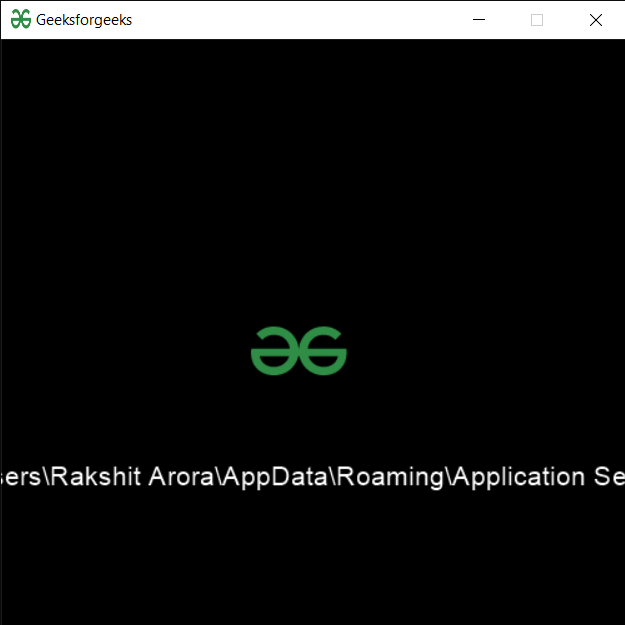# PYGLET – Getting Directory for Saving User Paths

• Last Updated : 06 Aug, 2021

In this article we will see how we can get the directory for saving paths of user in PYGLET module in python. Pyglet is easy to use but powerful library for developing visually rich GUI applications like games, multimedia etc. A window is a “heavyweight” object occupying operating system resources. Windows may appear as floating regions or can be set to fill an entire screen (fullscreen). In order to load a file i.e resource we use resource module of pyglet. This module allows applications to specify a search path for resources. Relative paths are taken to be relative to the application’s __main__ module. Different platforms have different conventions for where to save user preferences, saved games, and settings. This function implements those conventions. Note that the returned path may not exist: applications should use os.makedirs to construct it if desired. On Linux, a directory name in the user’s configuration directory is returned (usually under ~/.config). On Windows (including under Cygwin) the name directory in the user’s Application Settings directory is returned.
We can create a window object with the help of command given below

```# creating a window
window = pyglet.window.Window(width, height, title)```

In order to do this we use get_settings_path method with the pyglet.resource
Syntax : resource.get_settings_path(name)
Argument : It takes string as argument
Return : It returns string

Example: Below is the implementation

## Python3

 `#importing pyglet module``import` `pyglet``import` `pyglet.window.key as key``  ` `# width of window``width ``=` `500``  ` `# height of window``height ``=` `500``  ` `# caption i.e title of the window``title ``=` `"Geeksforgeeks"``  ` `# creating a window``window ``=` `pyglet.window.Window(width, height, title)``  ` `# text ``text ``=` `"Welcome to GeeksforGeeks"`` ` `# creating label with following properties``# font = cooper``# position = 250, 150``# anchor position = center``label ``=` `pyglet.text.Label(text,``                          ``font_name ``=``'Cooper'``,``                          ``font_size ``=` `16``,``                          ``x ``=` `250``, ``                          ``y ``=` `150``,``                          ``anchor_x ``=``'center'``, ``                          ``anchor_y ``=``'center'``)`  `# creating a batch``batch ``=` `pyglet.graphics.Batch()` `# loading geeksforgeeks image``image ``=` `pyglet.image.load(``'gfg.png'``)`  `# creating sprite object``# it is instance of an image displayed on-screen``sprite ``=` `pyglet.sprite.Sprite(image, x ``=` `200``, y ``=` `230``)``  ` `# on draw event``@window``.event``def` `on_draw():``      ` `    ``# clear the window``    ``window.clear()``      ` `    ``# draw the label``    ``label.draw()``    ` `    ``# draw the image on screen``    ``sprite.draw()``      ` `# key press event    ``@window``.event``def` `on_key_press(symbol, modifier):``  ` `    ``# key "C" get press``    ``if` `symbol ``=``=` `key.C:``        ` `        ``# printing the message``        ``print``(``"Key : C is pressed"``)``        ` `# image for icon``img ``=` `image ``=` `pyglet.resource.image(``"gfg.png"``)` `# setting image as icon``window.set_icon(img)`  `# getting directory setting path``value ``=` `pyglet.resource.get_settings_path(``"Application Settings"``)` `# setting text  of label``label.text ``=` `str``(value)` `# start running the application``pyglet.app.run()`

Output :My Personal Notes arrow_drop_up